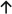# Term Deposit Calculator

See my savings

Total savings

--

Regular deposits

--

Total interest

--

-- -- -- -- -- YEAR REGULAR DEPOSITS INTEREST TOTAL SAVINGS TOTAL

Australia's Money Matchmaker™. The new, smarter way to compare. Take our quiz and we'll find you the best loan matches based on your answers.

Find my match## How to use the Term Deposit calculator

To use the term deposit calculator, you’ll need to enter some details about your deposit. These are explained below:

• Deposit Amount - This is the starting amount of money you plan to deposit into your term deposit. This will be the base amount any interest earnings is calculated on.
• Compound Frequency - How often interest is applied to your savings. Depending on the type of savings account you are using, this will be monthly or annually.
• Number of Years - With a term deposit, this will be the length of time you agree to when creating the term deposit.
• Interest Rate - This is the interest rate applied to your savings. For term deposits, there will be a fixed interest rate applied for the term.

Once you have entered the details about your estimated savings, you can click See My Savings to see how much you will gain through interest each year, including the total balance of your term deposit over the years.

## Term Deposit Interest Formula

The formula for compound interest on a single term deposit is: a = d ((1 + ( r / n )) ^ (n * p))

• a - the amount of money you will have at the end of the deposit period
• d - your initial deposit
• r - the annual interest rate expressed as a decimal
• n - the number of compounding periods per year - e.g. monthly = 12
• p - the number of years your money will be in savings and you will accrue interest

Keep in mind that this is a simple formula, and when calculating the compounding interest on an amount that includes a frequent deposit, you’ll need to alter the way you calculate the interest. You use the compound interest calculator to calculate interest for a savings account.

## Term Deposit Example Calculation

Let’s see how compound interest works in a simple example on a single term deposit. We’ll use a \$10,000 deposit earning 5% interest compounded monthly. You plan to leave the money untouched for 5 years.

• a = d ((1 + ( r / n )) ^ (n * p))
• a = 10000 * ((1 + (.05 / 12)) ^ (12*5))
• a = 10000 * (1.00416666667) ^ (60)
• a = 10000 * (1.28335867876) a = 12,833.59
• Total amount in savings = \$12,833.59
• Interest Earned is (a - d). In this example, that would be 12,833.59 - 10,000 = 2,833.59
• Interest Earned = \$2,833.59

## Example of Term Deposit Interest

Deposit amount2% interest4% interest5% interest

\$2,000

\$220

\$447

\$567

\$5,000

\$553

\$1,137

\$1,418

\$10,000

\$1,105

\$2,237

\$2,836

\$15,000

\$1,660

\$3,355

\$4,254

\$20,000

\$2,212

\$4,473

\$5,671

\$25,000

\$2,764

\$5,593

\$7,090

\$30,000

\$3,317

\$6,711

\$8,508

\$40,000

\$4,423

\$8,948

\$11,344

\$50,000

\$5,529

\$11,184

\$14,180

Term deposit interest examples are calculated using an initial deposit amount and no regular deposits, with interest compounded monthly for a term of five years.

## Need money to reach your goals?

FIND THE BEST LOAN RATES

Money Pty Ltd,
Suite 3, Level 2,
1 Taylor Street,
Moorabbin, VIC, 3189 Australia

#### Company

Money Pty Ltd trading as Money
ABN: 42 626 094 773
ACL: 528698
AFCA: 83955